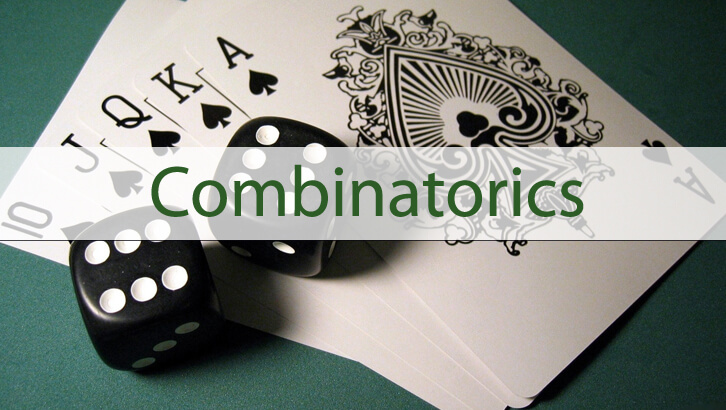# CAT Online Coaching – Permutation and Combination, Fixing the Errors II

This post had given a series of questions with incorrect solutions. Given below are the “debugged” solutions to questions 4, 5 and 6. The questions and the incorrect solutions here
4. In how many ways can we select 2 cards from a card pack such that both belong to the same suit?
Given solution
The first card can be selected in 52 ways, as it can be any card in the pack. Now, there are 12 different ways of selecting the second card. Depending on the suit of the first card, the choice set we have for the second card gets limited. If the first card were a club, then the second card must be from among the 12 remaining clubs and so on.
So, total number of outcomes = 52 * 12.
Bug in the solution:
We are double-counting when we do this. If we select 2H as the first card and 5D as the second card; this is same as selecting 5D as the first card and 2H as the second card. However, in our current approach, we will end up counting both of these.
Correct solution:
The correct approach would be to select one of the four suits first and then select 2 cards from 13 in that suit. Or, the number of ways of doing this = 4 * 13C2= 52 * 6.
5. When a coin is tossed 10 times, what is the probability of getting more heads than tails?
Given solution
When a coin is tossed ‘n’ times, the probability of getting more heads than tails = Probability of getting more tails than heads. So, we do not need to really compute this. Probability = 1/2.
Bug in the solution:
We are not counting the scenarios where there are an equal number of heads and tails. If we threw the coin an odd number of times, the probability of getting more heads than tails will be ½.
Correct solution:
Number of heads could be 6, 7, 8, 9, or 10. Probability = ( 10C6 + 10C7+ 10C8 + 10C9 + 10C10)/ 210
The other way of thinking about this would be ½ (1 – 10C5)/ 210. If we removed the scenario where the number of heads and tails are equal, the probability of more heads than tails should be equal to probability of more tails than heads.
6. In how many ways can we arrange 3 boys and 3 girls on a straight line such that no two boys stand next to each other

Given solution
We can arrange the 6 people as BGBGBG or GBGBGB. If we arranged them as BGBGBG, we would have 3! * 3! ways of arranging the 3 boys and the 3 girls. So, total number of possibilities = 2 * 3! * 3! = 72 ways.

Bug in the solution:
We are not accounting for arrangement such as BGBGGB and BGGBGB. Note that the question says that no two boys should stand next to each other. Two girls can stand next to each other.
Correct solution:
There are 4 possible outlines – BGBGBG, GBGBGB, BGBGGB or BGGBGB. So, total number of possibilities = 3! * 3! * 4 = 144.